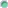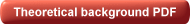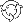The Product \ Summary

# Summary

## Short descriptionFind the most appropriate asset allocation according to your investment goals, market history and forecasts;Analyze risks of your investment portfolio from various perspectives (volatility, value-at-risk, shortfall probabilities);Arrive at adequate portfolio rebalancing strategy to minimize rebalancing transaction costs.

Supported analytical methods include shrinkage estimators, robust portfolio optimization, walk-forward portfolio optimization, benchmark tracking, Black-Litterman modelfactor models, and many others.## Features

Our advanced, constantly updated software gives the investor all the tools they need. Some of the mathematical algorithms utilized by SmartFolio have only emerged in the last few years. Many are therefore not to be found in other commercial products. The most important SmartFolio features are outlined below:

GeneralFully supports the multi-period investment paradigm.Fully supports portfolios featuring assets with non-Gaussian distribution of returns, or non-linear inter-dependencies, including options and hedge funds. This is achieved through direct simulation of portfolio dynamics with no model assumptions.

Portfolio ConstructionSimultaneous creation of two environments for portfolio analysis:   Analytical environment: logarithmic price increments are assumed to be independent normally distributed random variables. Historical environment: optimization and other procedures are based directly on historical prices.Risk-free asset option.Factor-selection option for a factor-based asset pricing model.

Estimation of parametersEqually-weighted sample estimates of expected returns and covariancesExponentially weighted sample estimates of expected returns and covariances (new in v.3.1)Stambaugh combined-sample estimates, used if asset histories differ in length. [pdf]Jorion expected returns estimate, which shrinks sample average returns to a common value.Ledoit-Wolf covariance matrix estimate, which shrinks the sample covariance matrix to the constant correlations covariance matrix. [pdf]Pastor-Stambaugh-Wang joint estimate of expected returns and covariances, which shrinks sample estimates to their respective counterparts, implied by the selected factor model. [pdf]MacKinlay-Pastor joint estimate of expected returns and covariances, based on the assumption that prices are explained by an unobservable factor. [pdf]The Black-Litterman model that incorporates subjective invetsor views in parameter estimation and asset allocation process. [pdf]Dummy estimates of expected returns and covariances further used in construction of risk-based portfolio strategies (risk parity and maximum diversification) (new in v.3.2)

Portfolio optimizationFour optimization criteria: Maximization of an expected utility with constant relative risk aversion Minimization of target shortfall probability [pdf] Benchmark tracking ( = volatility minimization relative to any benchmark asset) Maximizaton of instantaneous Sharpe ratio (utilized in construction of risk-based portfolio strategies) [pdf] (new in v.3.2)Robust portfolio optimization (worst-case scenario optimization): the resultant portfolios demonstrate optimal behavior under the worst-case scenario. [pdf]Walk-forward optimization: Arbitrary lengths of in-sample and out-of-sample windows Choice between rolling and expanding in-sample window (new in v.3.1)Optimization engine based on IPOPT (Internal Point OPTimizer) — one of the most powerful nonlinear optimizers available.

Target shortfall probabilities analysisCalculation of target shortfall probabilities according to selected ranges for the investment horizon and target rate.

Value-at-Risk analysisSimultaneous calculation of two risk measures: Value-at-Risk (VaR) and Conditional Value-at-Risk (CVaR).Various techniques for calculation of VaR and CVaR, including: Delta-Normal Method (DNM) Empirical distribution Implied normal distribution Implied non-central t-distribution [pdf] Cornish-Fisher expansion. [pdf]Construction of VaR and CVaR surfaces according to selected ranges for the investment horizon and significance level.

Historical simulationsSimulations of portfolio strategies with continuous rebalancing.Simulations of portfolio strategies with continuous rebalancing and portfolio insurance — these strategies are optimal in a situation when a predetermined portion of the initial wealth and/or accumulated profits must be maintained.Portfolio-strategy simulations with "inaction region" rebalancing — these strategies are optimal in the presence of proportional transaction costs.Portfolio-strategy simulations with "inaction region" rebalancing and portfolio insurance.

Data managementChoose either an Access-database or Excel spreadsheet format to store your data.Several historical data sources: Yahoo!Finance Text files Excel tables Bloomberg ProfessionalBatch import from all data sources1-click update from all data sources

Miscelaneous"Three-fund" portfolio calculation — utility-based portfolio, optimal in the presence of an estimation error in the model parameters. [pdf]Utilization of Block Bootstrapping algorithm in the calculation of VaR, CVaR, and shortfall probabilities.Determine Inaction region optimal size in the presence of proportional transaction costs, based on a multidimensional extension of the Davis-Norman approach. [pdf]Wide range of optimization constraints, which also include: Constraints on assets groups Highly non-linear margin constraint to account for margin requirements in portfolio components.Various performance measures including Information ratio, Sortino ratio and STARR ratio.